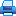Address: office 1410a, 17 Naberezhnaya Severnoy Dviny, Arkhangelsk, 163002, Russian Federation, Northern (Arctic) Federal University named after M.V. Lomonosov

Phone: (818-2) 21-61-21
E-mail: vestnik_est@narfu.ru
http://aer.narfu.ru/en/

# Checking Algorithm of Semilattice Isomorphism with the Use of Invariants of Graph Theory. P. 368–375Section: Physics. Mathematics. Informatics

519.178:512.53

### Authors

Larisa V. Zyablitseva*, Sergey A. Pestov*
*Northern (Arctic) Federal University named after M.V. Lomonosov (Arkhangelsk, Russian Federation)
Corresponding author: Larisa Zyablitseva, address: ul. Uritskogo, 68, korp. 3, Arkhangelsk, 163002, Russian Federation; e-mail: zlarisav@yandex.ru

### Abstract

Isomorphism of two commutative idempotent semigroups (semilattices) can be laid down by the algorithms of graph theory. For this, the graph is associated with the semilattices, and in the case when the resulting graph is a tree, known algorithms for checking tree isomorphism are used to verify the isomorphism of such semilattices. Another kind of graphs, which has a checking algorithm of isomorphism (differing from an exhaustive algorithm) are planar graphs. The article solves the question whether the graph of an arbitrary semilattice is a tree, a planar graph. We implement an algorithm to find out isomorphism of semilattices whose graphs are trees. This algorithm can be applied to arbitrary semilattices. In this case, for isomorphic semilattices the answer is true, and for nonisomorphic semilattices it can be erroneous. The paper demonstrates, which code word is given to an arbitrary semilattice, and the fact that this code word can serve as an invariant for checking the isomorphism of such a semilattice. We consider other invariants of graph theory that can be successfully applied to semilattices, and solve the question of completeness of the presented system of invariants. The resulting program for two arbitrary semilattices, given by Cayley tables, gives the information about the graphs (their invariants), determines their isomorphism. In the case of isomorphism, a bijective mapping of the elements of these semilattices is given. Using the program, the authors analyze all semigroups from the first to the eighth orders and find for each order the number of semilattices whose graphs are trees. The set of the proposed invariants for semilattices of no higher than eighth order is a complete system of invariants.

### Keywords

semigroup, graph, graph isomorphism, semigroup isomorphism, semilattice, tree, planar graph